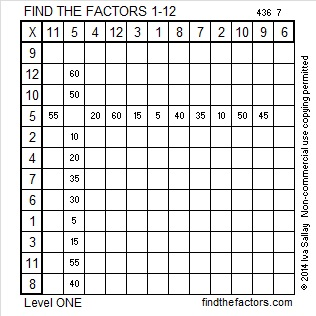# 226 and Level 1

• 226 is a composite number.
• Prime factorization: 226 = 2 x 113
• The exponents in the prime factorization are 1, and 1. Adding one to each and multiplying we get (1 + 1)(1 + 1) = 2 x 2 = 4. Therefore 226 has 4 factors.
• Factors of 226: 1, 2, 113, 226
• Factor pairs: 226 = 1 x 226 or 2 x 113
• 226 has no square factors so its square root cannot be simplified. √226 ≈ 15.033Excel file of puzzles and previous week’s factor solutions: 12 Factors 2014-09-08This site uses Akismet to reduce spam. Learn how your comment data is processed.